# ski-in / ski-out - Page 33

Viewing mode
743 results
• Slopes access :
• ski-in (-50 m)
• Rooms :
• 4 rooms
• Total surface (sq.m) :
• 70 sq.m
•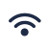•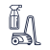•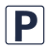•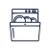•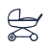•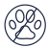• Slopes access :
• 50 -300m to the slopes
• Rooms :
• Studio + cabin(s)
• Total surface (sq.m) :
• 23 sq.m
••• Slopes access :
• 50 -300m to the slopes
• Rooms :
• Studio
• Total surface (sq.m) :
• 20 sq.m
•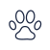••• Slopes access :
• 50 -300m to the slopes
• Rooms :
• 6 rooms
• Total surface (sq.m) :
• 100 sq.m
••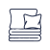•• Slopes access :
• ski-in (-50 m)
• Rooms :
• 2 rooms
• Total surface (sq.m) :
• 30 sq.m
••••• Slopes access :
• 50 -300m to the slopes
• Slopes access :
• 50 -300m to the slopes
• Rooms :
• Studio
• Studio + cabin(s)
• Total surface (sq.m) :
• 42 sq.m
•••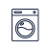••• Slopes access :
• ski-in (-50 m)
• Rooms :
• 4 rooms
• Total surface (sq.m) :
• 66 sq.m
••••• Slopes access :
• ski-in (-50 m)
• Rooms :
• Studio
• Studio + cabin(s)
• Total surface (sq.m) :
• 31 sq.m
••••• Slopes access :
• ski-in (-50 m)
• Total surface (sq.m) :
• 32 sq.m
•••••• Slopes access :
• 50 -300m to the slopes
• Rooms :
• Studio
• Studio + cabin(s)
• Slopes access :
• 50 -300m to the slopes
• Rooms :
• 4 rooms
• Total surface (sq.m) :
• 80 sq.m
•••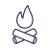••••• Slopes access :
• ski-in (-50 m)
• Rooms :
• 3 rooms
• Total surface (sq.m) :
• 60 sq.m
••••• Slopes access :
• ski-in (-50 m)
• Rooms :
• 3 rooms
• Total surface (sq.m) :
• 55 sq.m
•••••• Slopes access :
• ski-in (-50 m)
• Rooms :
• Studio + cabin(s)
• Total surface (sq.m) :
• 29 sq.m
••••• Slopes access :
• ski-in (-50 m)
• Rooms :
• 7 rooms
• Total surface (sq.m) :
• 120 sq.m
•••• Slopes access :
• 50 -300m to the slopes
• Rooms :
• 3 rooms
• Total surface (sq.m) :
• 60 sq.m
••••• Slopes access :
• ski-in (-50 m)
• Rooms :
• 2 rooms
• Total surface (sq.m) :
• 52 sq.m
••••• Slopes access :
• 50 -300m to the slopes
• Rooms :
• 3 rooms
• Total surface (sq.m) :
• de 60 à 80 sq.m
•••• Slopes access :
• ski-in (-50 m)
• Rooms :
• Studio
• Studio + cabin(s)
• Total surface (sq.m) :
• 30 sq.m
•••